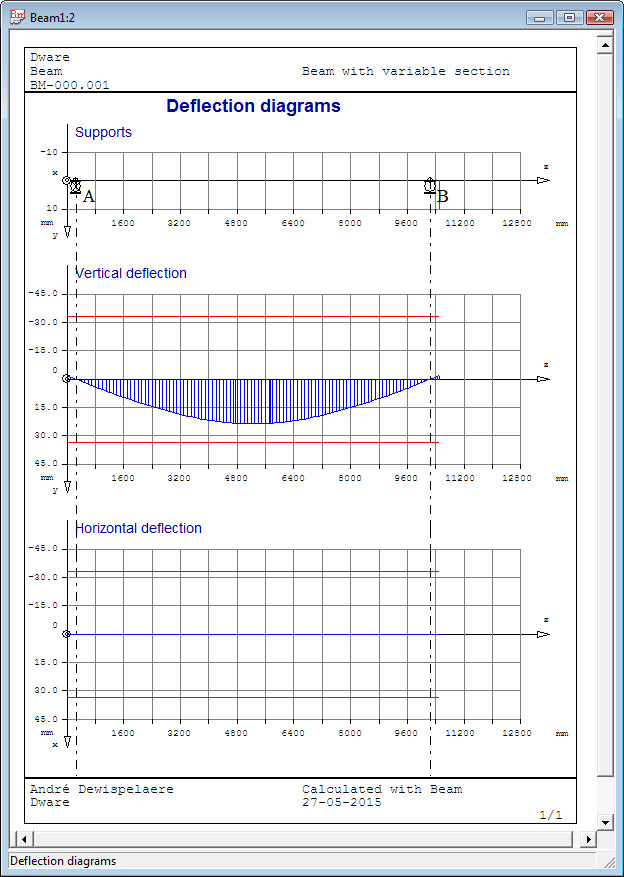### Calculation of Stresses

In the extreme vertices of a cross section, the bending stress is maximal, while the torsional shear and transverse shear are 0 or negligible small.
In the mid points of outer flanges and webs, the bending stresses are 0 or small, while the torsion shear and the transverse shear stresses are maximal.
Hence the vertex and mid points are examined separately.

Calculation of stress in four extreme vertex points:
bending stresses σz1,2,3,4 and normal stress σzn [N/mm²], comparison stress σcomp and the safety factor on failure SF.

Calculation of stress in the outer mid points:
bending stesses σz1,2,3,4 and normal stress σzn [N/mm²], torsional stress τt [N/mm²], shear stresses τsy and τsx, comparison stress σcomp and safety factor on failure SF.

Draw up of comparison stress diagram for both cases.

### Calculation of Deflections

The lateral deflections are calculated and presented in a table. Vertical deflection y and horizontal deflection x and twist angle φ. Example of vertical and horizontal deflection diagram:### Calculation of natural frequency

The natural or 'eigen' frequency is determined for the first bending mode.
The own weight of the beam and the distributed load (converted to a mass load) is taken into account for the mass-spring model. The distributed load is considered as a mass rigidly connected to the beam.

## Dimensioning Problem

Input of permissible stress σP [N/mm²] and the permissible deflection fP [mm].
Selection of a cross section with the Cross Section Switch dialog. See verification problem.

For a welded plate beam, the minimum required cross section is determined for the I - or box section to respect the stress - and deflection limits. In the dialog box the required input is displayed: b/h width/depth ratio, the flange thickness t and the web thickness s. The application iterates a calculation loop until the cross section respects the stress and deflection limits. Calculation results are displayed in a table: z, h, b, and b/h. The results table can be printed.